Author: Oscar Cronquist Article last updated on August 19, 2022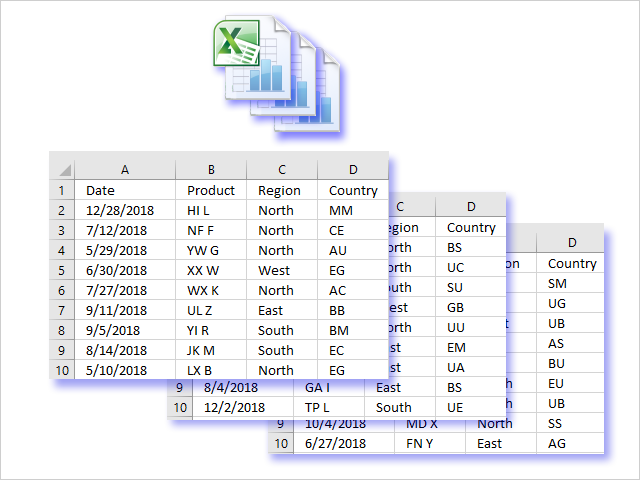I will in this article demonstrate a macro that automatically opens all workbooks in a folder and subfolders, one by one, and gets data from each sheet copied to a master workbook.

This allows you to quickly merge data across multiple workbooks saving you a lot of time and effort. To be able to consolidate data you need to make sure that data in each sheet begins in cell A1.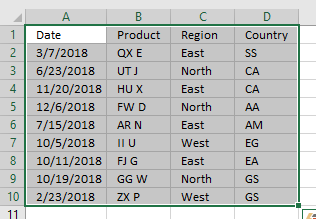The macro selects the current region based on cell A1 in each sheet, then copies the cell range to the master sheet in a new workbook.

For this to work the cells must be contiguous meaning there can't be blank rows or columns in the dataset.

The macro can, however, easily be modified to get a range based on the last non-empty cell in column A.

## 1. Macro VBA code

```'Name macro
Sub CopWKBooksInFolder()

'Dimension variables and declare data types
Dim WS As Worksheet
Dim myfolder As String
Dim Str As String
Dim a As Single
Dim sht As Worksheet

'Insert a new worksheet programmatically

'Show dialog box and prompt the user for a folder
With Application.FileDialog(msoFileDialogFolderPicker)
.Show
myfolder = .SelectedItems(1) & "\"
End With

'Dir function returns a string representing the name of a file or folder that matches a specified pattern.
Value = Dir(myfolder)

'Iterate through all files in folder
Do Until Value = ""

'Check that file name is not . (dot) or .. (two dots)
If Value = "." Or Value = ".." Then

'Continue here if file name is not . (dot) or .. (two dots)
Else

'Check if file extension matches xls, xlsx or xlsm
If Right(Value, 3) = "xls" Or Right(Value, 4) = "xlsx" Or Right(Value, 4) = "xlsm" Then

'Enable error handling
On Error Resume Next

'Check if file is password protected

'Check if an error has occurred
If Err.Number > 0 Then

'Continue here if an error has not occureed
Else

'Disable error handling
On Error GoTo 0

'Iterate through each worksheet in active workbook
For Each sht In ActiveWorkbook.Worksheets

'Check if first cell A1 is not empty
If sht.Range("A1") <> "" Then

'Find last non-empty cell in column A
Lrow = WS.Range("A" & Rows.Count).End(xlUp).Row + 1

'Copy cells
sht.Range("A1").CurrentRegion.Copy Destination:=WS.Range("A" & Lrow)
End If

'Continue with the next worksheet
Next sht
End If

'Close active workbook
Workbooks(Value).Close False

'Disable error handling
On Error GoTo 0
End If
End If
Value = Dir

'Continue with next file
Loop

Cells.EntireColumn.AutoFit
End Sub
```

## 2. Where to copy the code?

1. Copy above macro
2. Go to VBA Editor (Alt+F11)
3. Press with left mouse button on "Insert" on the top menu
4. Press with left mouse button on "Module" to insert a module to your workbook
5. Paste code into the code window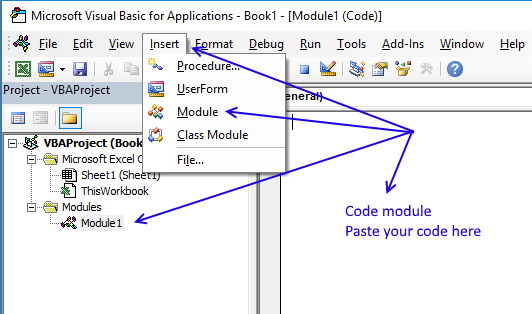## 3. Save the workbook

To be able to use the macro next time you open your workbook you need to save the workbook as a macro-enabled workbook.

1. Press with left mouse button on "File" on the menu, or if you have an earlier version of Excel, press with left mouse button on the office button.
2. Press with left mouse button on "Save As"
3. Press with left mouse button on file extension drop-down list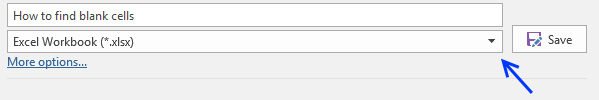4. Change the file extension to "Excel Macro-Enabled Workbook (*.xlsm)".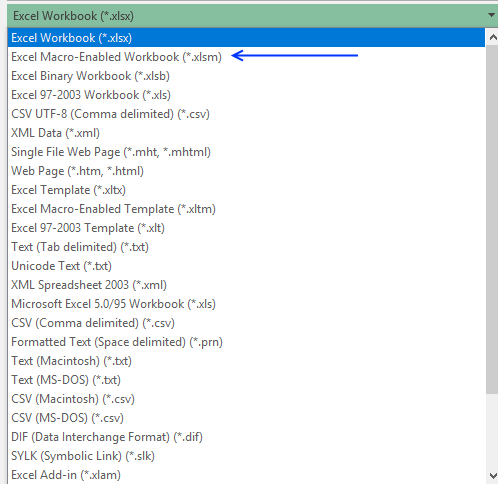## 4. How to use the macro?

1. Open the Macro dialog box (Alt + F11)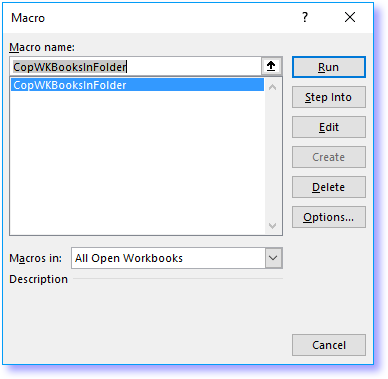2. Select CopWKBooksInFolder.
3. Press with left mouse button on "Run" button.
4. A folder dialog box appears.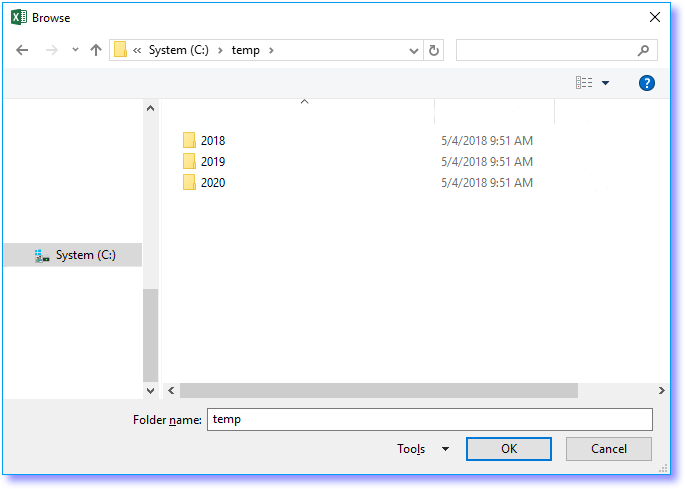5. Navigate to a folder you want to search.
6. Press with left mouse button on OK button.
7. The macro starts opening workbooks, one by one, copying values to a master worksheet.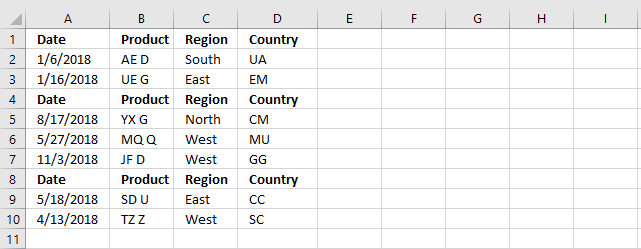The picture above demonstrates a master worksheet, even the headers are copied to the worksheet.

## 5. Copy data ignore headers

The following macro copies all data from the first opened workbook and worksheet, worksheets after that ignores the header row.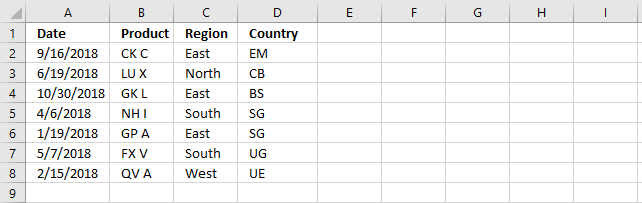There is only one header row in the picture above.

```Sub CopWKBooksInFolder()
Dim WS As Worksheet
Dim myfolder As String
Dim Str As String
Dim a As Single
Dim sht As Worksheet

With Application.FileDialog(msoFileDialogFolderPicker)
.Show
myfolder = .SelectedItems(1) & "\"
End With
chk = 0
Value = Dir(myfolder)
Do Until Value = ""
If Value = "." Or Value = ".." Then
Else
If Right(Value, 3) = "xls" Or Right(Value, 4) = "xlsx" Or Right(Value, 4) = "xlsm" Then
On Error Resume Next
If Err.Number > 0 Then
Else
On Error GoTo 0
For Each sht In ActiveWorkbook.Worksheets
If sht.Range("A1") <> "" Then
Lrow = WS.Range("A" & Rows.Count).End(xlUp).Row + 1
If chk = 0 Then
sht.Range("A1").CurrentRegion.Copy Destination:=WS.Range("A" & Lrow)
chk = 1
Else
Set crng = sht.Range("A1").CurrentRegion
Set crng = crng.Offset(1, 0)
Set crng = crng.Resize(crng.Rows.Count - 1)
crng.Copy Destination:=WS.Range("A" & Lrow)
End If
End If
Next sht
End If
Workbooks(Value).Close False
On Error GoTo 0
End If
End If
Value = Dir
Loop
Cells.EntireColumn.AutoFit
End Sub
```

## 6. Copy data from specific worksheets in folders based on paths

This macro ignores header rows except the first one, as well. It also allows you to copy data from worksheets whose names contain a specific text string.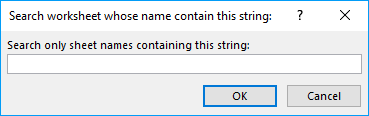The the macro allows you to select a cell range containing search paths to folders you want to search.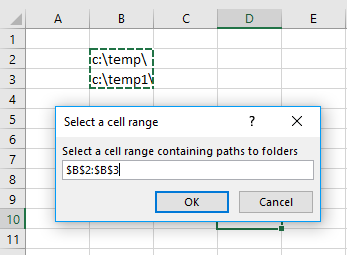```Sub CopWKBooksInFolder()
Dim WS As Worksheet
Dim myfolder As String
Dim Str As String
Dim a As Single
Dim sht As Worksheet

Str = Application.InputBox(prompt:="Search only sheet names containing this string:", Title:="Search worksheet whose name contain this string:", Type:=2)

On Error Resume Next
Set Rng = Application.InputBox(prompt:="Select a cell range containing paths to folders" _
, Title:="Select a cell range", Default:=ActiveCell.Address, Type:=8)
On Error GoTo 0

For Each cell In Rng

If Dir(cell.Value, vbDirectory) <> "" Then

chk = 0
Value = Dir(cell.Value)

Do Until Value = ""
If Value = "." Or Value = ".." Then
Else
If Right(Value, 3) = "xls" Or Right(Value, 4) = "xlsx" Or Right(Value, 4) = "xlsm" Then
On Error Resume Next
If Err.Number > 0 Then
Else
On Error GoTo 0
For Each sht In ActiveWorkbook.Worksheets

If InStr(sht.Name, Str) <> 0 Then

If sht.Range("A1") <> "" Then
Lrow = WS.Range("A" & Rows.Count).End(xlUp).Row + 1

If chk = 0 Then
sht.Range("A1").CurrentRegion.Copy Destination:=WS.Range("A" & Lrow)
chk = 1
Else
Set crng = sht.Range("A1").CurrentRegion
Set crng = crng.Offset(1, 0)
Set crng = crng.Resize(crng.Rows.Count - 1)
crng.Copy Destination:=WS.Range("A" & Lrow)
End If
End If
End If
Next sht
End If
Workbooks(Value).Close False
On Error GoTo 0
End If
End If
Value = Dir
Loop
End If
Next cell
Cells.EntireColumn.AutoFit
End Sub
```